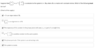# Which statements are true given a solution to the system x'=Ax?

• JessicaHelena

#### JessicaHelena

Summary:: Suppose that [x, y] = e^{-3t} [-2, -1] is a solution to the system $x' = Ax$, where A is a matrix with constant entries. Which of the following must be true?

a. -3 is an eigenvalue of A.
b. [4, 2] is an eigenvector of A.
c. The trajectory of this solution in the phase plane with axes x, y is part of a straight line.
d. e^{-3t} [-1, -2] is another solution to the same system.
e. the phase portrait of the system is an attracting node.
f. the system is stable.

Please refer to the attached screenshot for clearer question; however, the checked answers there are not my final answers.

This is a star node, and hence ##\lambda = -3##. (Hence ##a## is right.) Since the eigenvalue is negative, it is an attracting node (which makes ##e## correct).
I graphed the parametric function, and the result was a straight line extending only in the 3rd quadrant. Hence ##c## is right.
I wasn't sure of a good mathematical way to get the matrix A, but by trial and error, got that A = [0, -6; -3, 3]. Checking for ##Av = \lambda v##, I got the right answer, which makes me believe I have the right A. From this, trA = 3, and det A = -18. Checking against the rule of stability, we get that this system is not stable. (hence ##f## is not an answer).

I am not sure how exactly to do b and d, but using the rule that ##Av = \lambda v##, I don't believe they are right.

Hence I would check a, c, and e. Is this way of thinking right?

[Moderator's note: Moved from a technical forum and thus no template.]

#### Attachments

•Screenshot 2020-02-08 at 5.22.32 PM.png
16.1 KB · Views: 131
Last edited by a moderator:

Since the eigenvalue is negative, it is an attracting node (which makes eee correct).
What about the other eigenvalues of A?

I wasn't sure of a good mathematical way to get the matrix A, but by trial and error, got that A = [0, -6; -3, 3].
That would be because there is no mathematical way. You do not have enough information to determine A.

I am not sure how exactly to do b and d, but using the rule that Av=λvAv=λvAv = \lambda v, I don't believe they are right.
Is [-2,-1] an eigenvector of A?

@Orodruin

What about the other eigenvalues of A?
I had thought that this was a repeated eigenvalue, since the second constant c2 = 0.
Is [-2,-1] an eigenvector of A?
I had thought that the way solutions are formatted is
[x, y] = c_1 e^{##\lambda_1 t##} *eigenvector_1 + c_2 e^{##\lambda_2 t##}*eigenvector_2.
From this, I thought that c_2 = 0 (which led me to the conclusion that this was a star node) and that [-2, -1] was an eigenvector of A.

I had thought that this was a repeated eigenvalue, since the second constant c2 = 0.
It just says that this is a solution. A priori, this does not tell you anything about the other eigenvalues.

I had thought that the way solutions are formatted is
[x, y] = c_1 e^{##\lambda_1 t##} *eigenvector_1 + c_2 e^{##\lambda_2 t##}*eigenvector_2.
From this, I thought that c_2 = 0 (which led me to the conclusion that this was a star node) and that [-2, -1] was an eigenvector of A.

Yes, ##c_2## is zero, that is why you know nothing about the second eigenvalue. However, it is correct that [-2 -1] is an eigenvector. Are [4 2] and [-2 -1] linearly independent?

@Orodruin

is zero, that is why you know nothing about the second eigenvalue. However, it is correct that [-2 -1] is an eigenvector. Are [4 2] and [-2 -1] linearly independent?
Well, yes, which actually makes b true as well.

Hmm, so. So far I know that

a. -3 is an eigenvalue of A.
b. [4, 2] is an eigenvector of A.
are right.

c. The trajectory of this solution in the phase plane with axes x, y is part of a straight line.
should be right because I graphed the parametric function.

But since I don't know the matrix A, and there isn't a surefire way to figure out one (from what I gather),

d. e^{-3t} [-1, -2] is another solution to the same system.
e. the phase portrait of the system is an attracting node.
f. the system is stable.

will not necessarily be right because
d: we don't know A, so I cannot really use ##Av = \lambda v## to check
e: While negative eigenvalues do make attracting node, if the system has another eigenvalue that is non-negative, the phase portrait wouldn't be an entirely attracting node
f. I need that trA < 0 and detA > 0 for the system to be stable, but I don't know A.

Is this way of thinking right?

Looks ok to me.

Yes—that turned out to be right. Thank you!